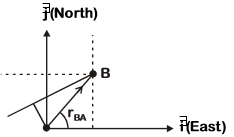# Ship A is sailing towards north−east with velocity= 30+ 50km/hr wherepoints east and, north.  Ship B is at a distance of 80 km\east and 150 km north of Ship A and is sailing towards west at 10 km/hr. A will be at minimum distance from B in (a) 2.2 hrs. (b) 4.2 hrs. (c) 3.2 hrs (d) 2.6 hrs.

## Question ID - 51160 :- Ship A is sailing towards north−east with velocity= 30+ 50km/hr wherepoints east and, north.  Ship B is at a distance of 80 km\east and 150 km north of Ship A and is sailing towards west at 10 km/hr. A will be at minimum distance from B in (a) 2.2 hrs. (b) 4.2 hrs. (c) 3.2 hrs (d) 2.6 hrs.

3537A=30+50km/hrBA =(80+ 150)kmB =(−10)km/hrBA=BA=−10−30=−40−50Projection of

(onBA===∴t===2.6hrs

Next Question :
 For the circuit shown, with R1 = 1.0, R2 = 2.0, E1 = 2 V and E2 = E3 = 4 V, the potential difference between the points ‘a’ and ‘b’ is approximately (in V)(a) 2.7 (b) 3.7 (c) 2.3 (d) 3.3